The best tools to make your project dreams come true

# How to Control a DC Fan Using the Raspberry Pi

## 2/27/2019 | By Maker.io Staff

Previously, we learned how to control external devices using the Raspberry Pi, basic circuitry, and Python. In this article, we will learn how to control a DC fan using the Pi and Python!

### BOM

• Raspberry Pi 3 Model B+
• IRF830
• 10k Resistor
• 12V DC fan
• ### Scheme-It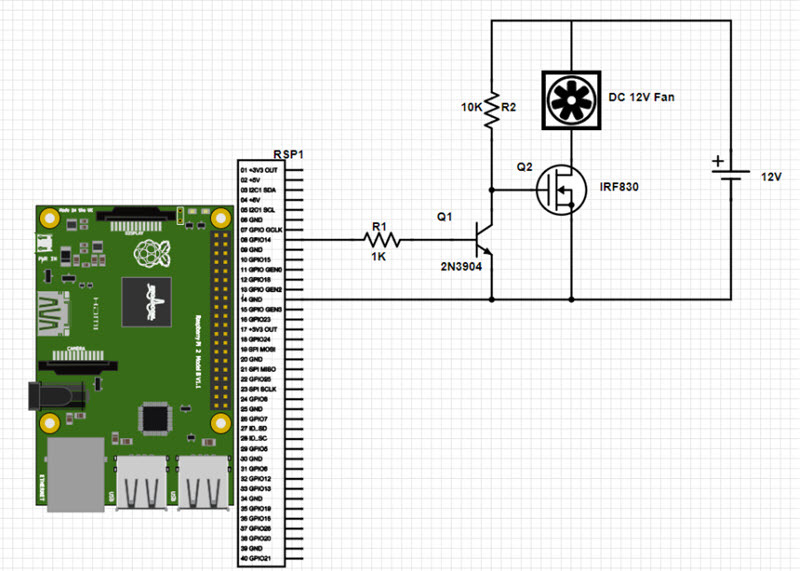https://www.digikey.com/schemeit/project/dcfancontrol-KE1VU2G30060/

### PWM: Pulse Width Modulation

Fans are useful devices that create airflow to cool something down, provide a fresh supply of air, or help the circulation of aerosols. Most domestic fans have different speed settings, but these are often in the form of mechanical buttons, which may be difficult to control using a Raspberry Pi. Sure, a circuit that has a servo and an arm could be used to press buttons, but that’s a rather complex solution. Instead, it is easier to control how much power the fan is allowed to consume and utilizing PWM signals allows the system to have complete control over the fan speed.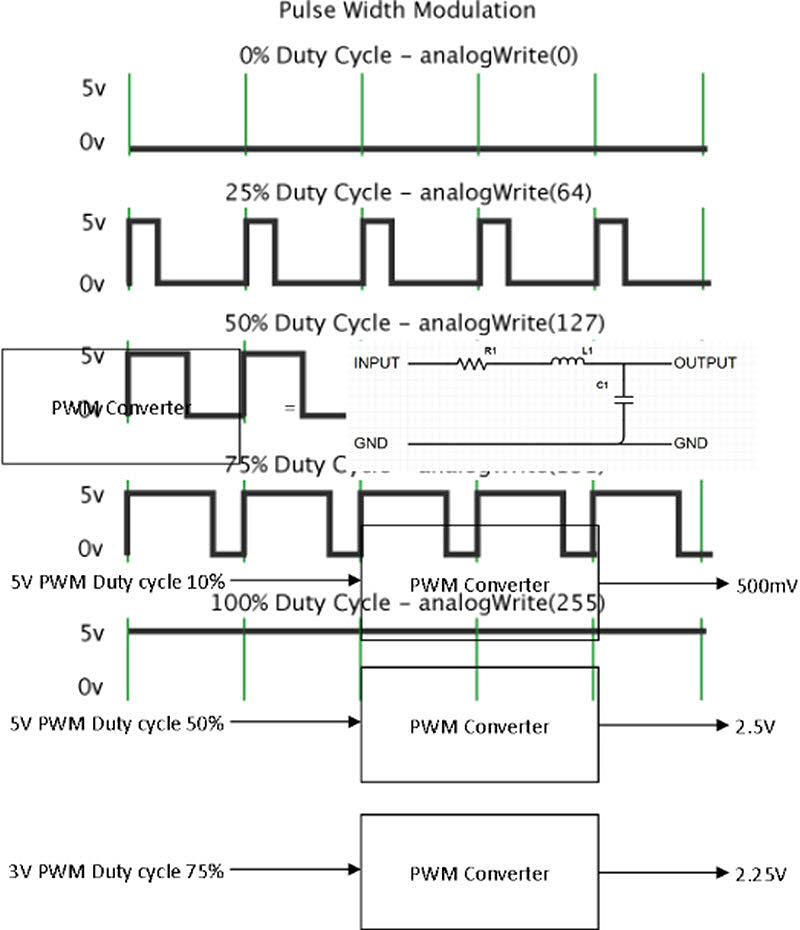PWM signals are square waves that have arbitrary on and off lengths. Whereas a perfect square wave has the on time of the signal the same length as the off signal. What makes PWM signals useful in the field of power control is if a PWM signal is averaged using a filter, the result is an analog voltage. This voltage is considered analog, as the value of the voltage is proportionate to the duty cycle of the original PWM signal. It is easier to understand this using some simple examples.

This equation is used to calculate the output voltage of an averaged PWM signal: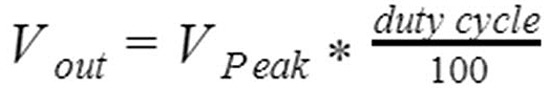While it may seem complex, consider this simplified explanation. PWM signals on their own cannot be used to control power to every electrical device (such as computers and TVs). They can, however, control the power of devices such as motors and light bulbs without the need of a PWM converter. Therefore, how can we use PWM signals to control the speed of a DC fan? Our simple solution includes a MOSFET, BJT, and 2 resistors to do it!

### How It Works: The Circuit

PWM signals are sent out of GPIO14 using the Python script (described below) which results in the transistor Q1 turning on and off. When the transistor Q1 is on, then the effective resistance of Q1 is 0Ω and therefore the voltage at the gate of Q2 is also 0V. Since the Gate-Source voltage on Q2 is now 0V, then Q2 turns off and prevents the 12V fan from being able to draw any current. When the output of GPIO14 turns off, then transistor Q1 also turns off and results in the gate voltage of Q2 being 12V. Because the Gate-Source voltage of Q2 is now 12V, the transistor Q2 fully conducts and enables the fan to turn on.

If the PWM signal from GPIO14 had a very low frequency - turning on and off once every few seconds - then the fan would speed up fully, then stop moving, then speed up, then stop moving again. However, the frequency of the PWM signal is in the hundreds of hertz and this results in the PWM signal to the fan being smoothed and averaged. Therefore, the speed of the fan will be roughly proportional to the input PWM duty cycle. As the duty cycle increases, then the speed of the fan also increases.

### How It Works: The Software

The software for PWM DC control is very simple and uses the library RPi.GPIO. The first two lines of the code import all the needed libraries which include the time library (for making delays) and the GPIO library. The GPIO library is configured to use the BCM numbering scheme and GPIO 14 is configured as an output.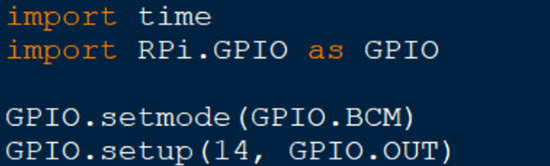The next two lines configure GPIO 14 as a PWM output, create a PWM object called pwmOut, and start the PWM signal. The .PWM() function takes two arguments; the first argument defines which pin is being used as the PWM output, and the second argument determines the frequency of the PWM signal. In this program, we set the PWM signal to a frequency of 200Hz.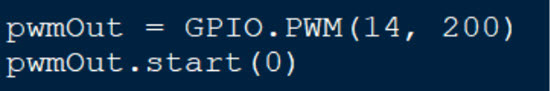The main program loop sets the value of the duty cycle variable and then changes the duty cycle of the PWM signal using the .ChangeDutyCycle() function. The reason why the value is duty cycle variable is subtracted from 100 is due to the use of the 2N3904. The 2N3904 is in an inverting configuration, which means when we want to turn the fan on, we need to turn off the 2N3904.

import time

import RPi.GPIO as GPIO

GPIO.setmode(GPIO.BCM)

GPIO.setup(14, GPIO.OUT)

pwmOut = GPIO.PWM(14, 200)

pwmOut.start(0)

# Remember, the fan is connected to an inverter (BJT)

# so the duty cycle is the opposite ;)

dutyCycle = 0

# Main program loop

while(1):

time.sleep(0.2)

dutyCycle = dutyCycle + 1

if(dutyCycle > 100):

dutyCycle = 0

pwmOut.ChangeDutyCycle(100 - dutyCycle)